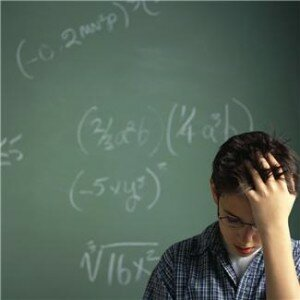# Basic Algebra Practice Test QuestionsBasic Algebra questions are common on most College Entrance exams, High School exams, Nursing Entrance Exams and High School Equivalence exams.

# Algebra Practice Test Questions

1. It is known that x2+4x=5. Then x can be

1. 0
2. -5
3. 1
4. Either (b) or (c)

2. (a+b)2 = 4ab . What is necessarily correct?

1. a>b
2. a<b
3. a=b
4. None of the Above

3. Sum of the digits of a 2-digit number is 12. If we switch the digits, the number we get will be greater than the initial one by 36. Find the initial number

1. 39
2. 48
3. 57
4. 75

4. Two friends traveled to a nearby city. In the second day they travelled 75 miles more than the first day, and in the third day, they travelled a third of the distance covered in the second day. How many miles did they cover in the first day, if the total travelled was 170 miles?

1. 30 miles
2. 35 miles
3. 105 miles
4. 135 miles

5. Kate’s father is 32 years older than Kate. In 5 years he will be five times older than his daughter. How old is Kate?

1. 2
2. 3
3. 5
4. 6

6. If Lynn can type a page in p minutes, what portion of the page can she do in 5 minutes?

1. 5/p
2. p – 5
3. p + 5
4. p/5

7. If Sally can paint a house in 4 hours, and John can paint the same house in 6 hours, how long will it take for both of them to paint the house together?

1. 2 hours and 24 minutes
2. 3 hours and 12 minutes
3. 3 hours and 44 minutes
4. 4 hours and 10 minutes

8. Employees of a discount appliance store receive an additional 20% off of the lowest price on any item. If an employee purchases a dishwasher during a 15% off sale, how much will he pay if the dishwasher originally cost \$450?

1. \$280.90
2. \$287
3. \$292.50
4. \$306

1. D
x2 +4x=5, x2+4x-5=0, x2+5x-x-5=0, factorize x(x+5)-1(x+5)=o, (x+5)(x-1)=0.  x+5=0 or x-1=0, x=0-5 or x=0+1,  x= -5 or x=1, either b or c

2. C
Open parenthesis: 2a + 2b = 4ab, divide both sides by 2 = a+b=2ab or a+b=ab + ab, therefore a=ab and b=ab, therefore a=b

3. B
Let the XY represent the initial number, X + Y = 12, YX=XY+ 36, Only b=48 satisfies both equations above from the given options

4. A

5. B

Let the father’s age=Y, and Kate’s age=X, therefore Y=32+X, in 5yrs y=5x,substituting for Y will be 5x = 32+X, 5x – x = 32, 4X=32,X= 32/8, x = 8, Kate will be 8 in 5 yrs time, so Kate’s present age = 8-5=3

6. D

7. A

Let X represent the house, Sally paints X in 4hrs or ¼ X per 1hr or 60 minutes, John paints X in 6 hours or at 1/6X per 1hr or 60mins. Working together, they will paint 1/4x + 1/6x in 1hr or 60minutes = 10/24x = 5/12x every 60 minutes, to paint x = 60 minutes x 12/5 = 144 minutes or 2 hrs and 24 minutes

8. D

Cost of dishwasher = \$450, 15% discount = 15/100 x 450 = \$67.5, new price = 450 – 67.5 = \$382.5, 20% discount on lowest price = 20/100 x 382.5 = \$76.5, final price = \$306
Algebra tutorial at Wikipedia